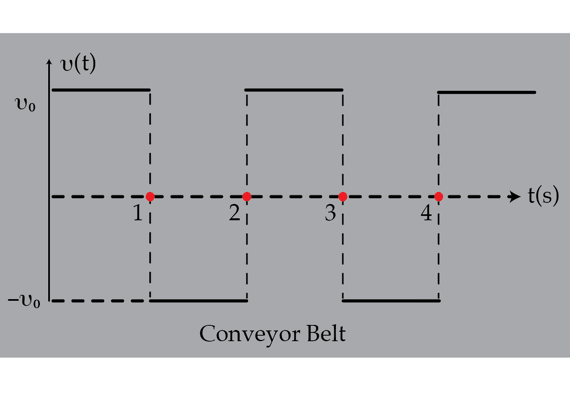# Faulty conveyor belt

A small box is placed on a conveyor belt that moves with constant speed $v_{0}=1~\text{m}/\text{s}$. Due to a technical malfunction, the belts starts moving left and right at constant speed $v_{0}$ (see the graph below). The coefficient of kinetic friction when the box moves to left, relative to the belt, is $\mu_{L}=0.4$ and it is $\mu_{R}=0.3$ when the box moves to the right, relative to the belt. As a result, of this frictional anisotropy, the box moves, on average, to the right. Assume that the conveyor belt is very long, determine the magnitude of the terminal average velocity in meters per second of the box (in the laboratory frame of reference).Details and assumptions

Assume that $g=9.8~\text{m}/\text{s}^{2}$.

×

Problem Loading...

Note Loading...

Set Loading...